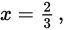# SAT Math Multiple Choice Question 324: Answer and Explanation

### Test Information

Question: 324

9. How many real values of x satisfy the quadratic equation 9x2 – 12x + 4 = 0?

• A. 0
• B. 1
• C. 2
• D. 4

Getting to the Answer: Notice that the first and last terms in the equation are perfect squares-this is a hint that it could be a perfect square trinomial, which it is. The factored form of the equation is (3x - 2)(3x - 2). Both factors are the same, so there is only one real value,that satisfies the equation.